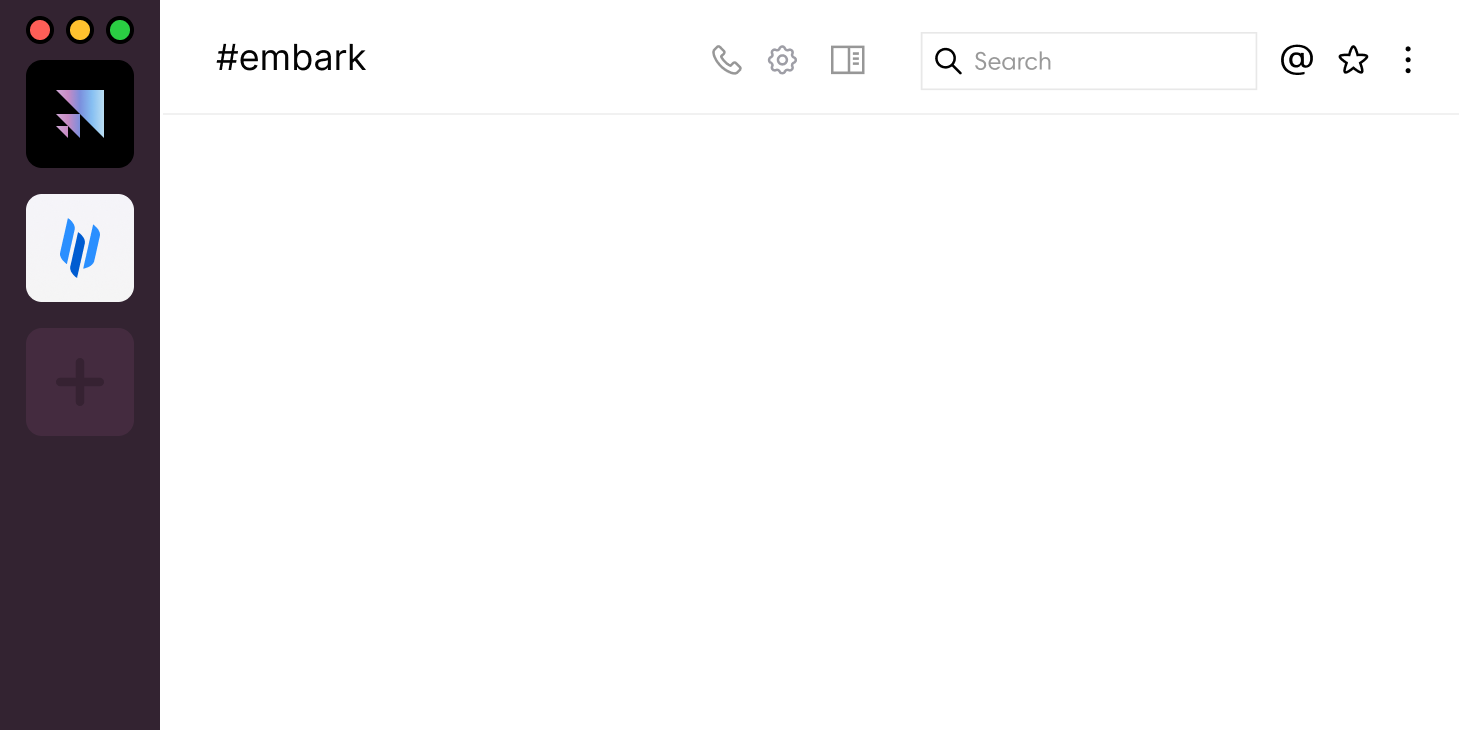•••••••# A Comprehensive Set of Tools self.__wrap_b=(t,n,e)=>{e=e||document.querySelector(`[data-br="\${t}"]`);let s=e.parentElement,r=R=>e.style.maxWidth=R+"px";e.style.maxWidth="";let o=s.clientWidth,i=s.clientHeight,c=o/2-.25,l=o+.5,u;if(o){for(;c+1<l;)u=Math.round((c+l)/2),r(u),s.clientHeight===i?l=u:c=u;r(l*n+o*(1-n))}e.__wrap_o||(e.__wrap_o=new ResizeObserver(()=>{self.__wrap_b(0,+e.dataset.brr,e)})).observe(s)};self.__wrap_b(":R15iadb76:",0.4)

Pixel Perfection

Multiple Classes

Internationally Versatile

# Custom Annual Plans and SLAs self.__wrap_b=(t,n,e)=>{e=e||document.querySelector(`[data-br="\${t}"]`);let s=e.parentElement,r=R=>e.style.maxWidth=R+"px";e.style.maxWidth="";let o=s.clientWidth,i=s.clientHeight,c=o/2-.25,l=o+.5,u;if(o){for(;c+1<l;)u=Math.round((c+l)/2),r(u),s.clientHeight===i?l=u:c=u;r(l*n+o*(1-n))}e.__wrap_o||(e.__wrap_o=new ResizeObserver(()=>{self.__wrap_b(0,+e.dataset.brr,e)})).observe(s)};self.__wrap_b(":R15iqdb76:",0.4)

• ### Guaranteed Task Completion Time

Each enterprise customer is paired with a dedicated engagement manager who will ensure smooth on-boarding and continued data delivery.• ### Cost Effective

Enterprise engagements provide upfront and volume-based discounts, and is the most cost-effective solution for high-quality labels. Plus with Scale AI, there are no platform fees.

# Best-In-Class Quality self.__wrap_b=(t,n,e)=>{e=e||document.querySelector(`[data-br="\${t}"]`);let s=e.parentElement,r=R=>e.style.maxWidth=R+"px";e.style.maxWidth="";let o=s.clientWidth,i=s.clientHeight,c=o/2-.25,l=o+.5,u;if(o){for(;c+1<l;)u=Math.round((c+l)/2),r(u),s.clientHeight===i?l=u:c=u;r(l*n+o*(1-n))}e.__wrap_o||(e.__wrap_o=new ResizeObserver(()=>{self.__wrap_b(0,+e.dataset.brr,e)})).observe(s)};self.__wrap_b(":R15ljadb76:",0.4)

### Super Human Quality

Autonomous Driving tasks submitted to the platform are first pre-labeled by our proprietary ML-model, then manually reviewed by highly trained workers depending on the ML model confidence scores. All tasks receive additional layers of both human and ML-driven checks.

The resulting accuracy is consistently higher than what a human or synthetic labeling approach can achieve independently.# Trusted by World Class Companies self.__wrap_b=(t,n,e)=>{e=e||document.querySelector(`[data-br="\${t}"]`);let s=e.parentElement,r=R=>e.style.maxWidth=R+"px";e.style.maxWidth="";let o=s.clientWidth,i=s.clientHeight,c=o/2-.25,l=o+.5,u;if(o){for(;c+1<l;)u=Math.round((c+l)/2),r(u),s.clientHeight===i?l=u:c=u;r(l*n+o*(1-n))}e.__wrap_o||(e.__wrap_o=new ResizeObserver(()=>{self.__wrap_b(0,+e.dataset.brr,e)})).observe(s)};self.__wrap_b(":Rj5jqdb76:",0.4)

••••••••••••••••••••“Very quickly, our engineers liked what they saw and we asked Scale to ramp 10X throughput in a matter of weeks. Scale’s been able to support the extra throughput request and allowed us to do great research.”

#### David Garber

Product Manager, TRI“Scale is basically providing the ground truth for our perception systems. It’s a very, very critical piece for us to develop.”

#### Anantha Kancherla

Head of self driving software, Lyft

## Get Labeled Data Today!